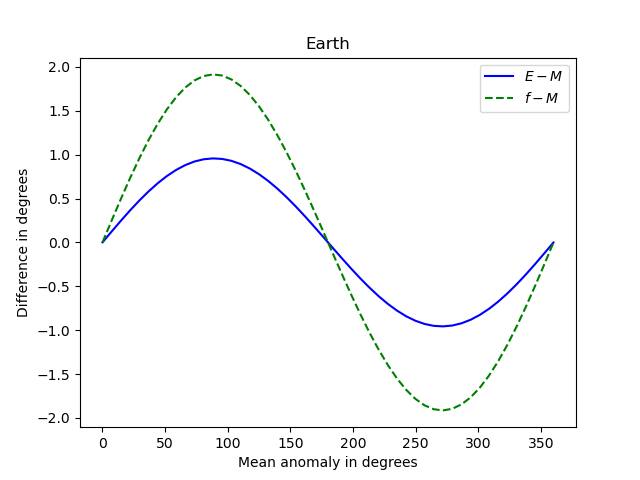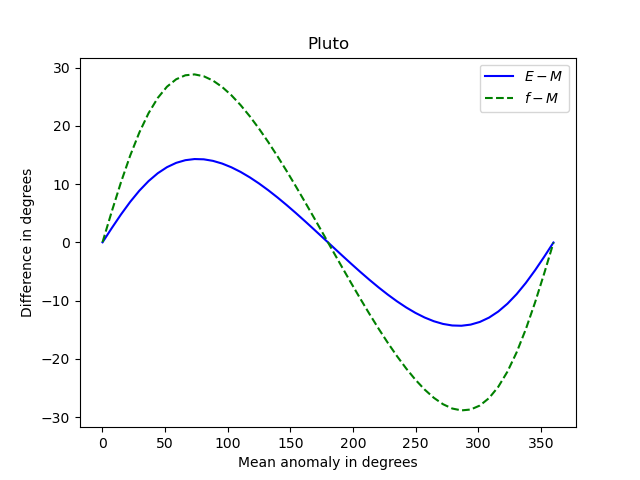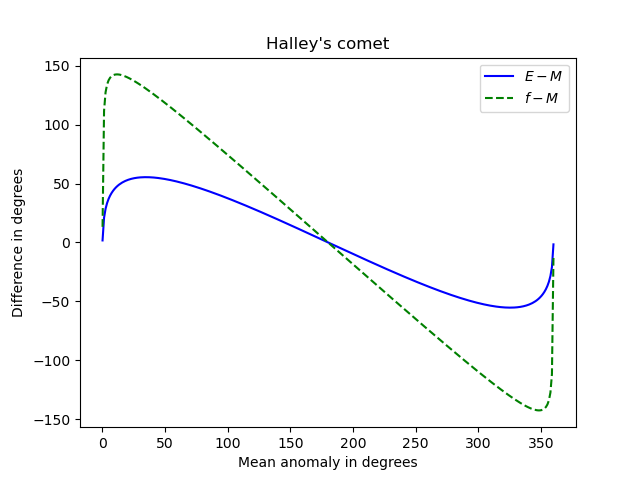# Mean anomaly, true anomaly, and eccentric anomaly

Orbital mechanics has a lot of arcane terminology because it has been studied for centuries. V. I. Arnold said that orbital mechanics was one of the three main sources of modern mathematics.

Mean anomaly, true anomaly, and eccentric anomaly are three ways of describing where an object is in its orbit. All would be the same if planets moved in circular orbits. And since planets nearly do move in circular orbits , at least in our solar system, these three anomalies will be similar. We will demonstrate this with plots.

## Mean anomaly

Suppose a planet orbiting a star has period T, i.e. it takes time T for the planet to complete one orbit. Let t0 be the time when the planet was closest to the star, i.e. the time since the planet passed through periapsis . Let t be the current time. Then the mean anomaly M is simply

M = 2π(tt0) / T

if you’re working in radians. Change 2π to 360° if you’re working in degrees.

## True anomaly

The true anomaly f is the angle with vertex at the star and sides running from the star to periapsis and from the star to the planet. (I don’t know why true anomaly is denoted f. I suppose t and T were taken.)

## Eccentric anomaly

The eccentric anomaly E is sorta like the true anomaly, but with the vertex at the center of the elliptical orbit rather than at the star. I say sorta because that’s not quite right.

The eccentric anomaly is an angle with vertex at the center of the elliptical orbit. (The star is not at the center because elliptical orbits are eccentric, literally off-center.) One side does run along the major axis as with the true anomaly, but the other side doesn’t extend from the center to the planet but rather from the center to a projection of the planet’s orbit onto a circle.

Imagine an elliptical orbit in the plane, centered at the origin, with major axis along the x-axis. Now draw a circle around the ellipse with radius equal to the semi-major axis of the ellipse. So the circle touches the ellipse on the two ends, apsis and periapsis, but otherwise extends above and below the ellipse. Take a point P in the orbit and push it out vertically to a point P‘ on the circumscribed circle. That is, keep the x coordinate of P the same, but push the y coordinate up if it’s positive, or down if it’s negative, to get a point on the circle.

## Equations

Kepler’s equation relates mean anomaly and eccentric anomaly:

M = Ee sin E

where M is the mean anomaly, E is the eccentric anomaly, and e is the eccentricity of the orbit. So when e is small, M is approximately equal to E.

True anomaly f is related to eccentric anomaly by

f = E + 2 arctan( β sin E / (1 – β cos E) )

where

β = e / (1 + √(1 – e²)).

I’ve written about Kepler’s equation a couple times. Here is a post about a simple way to solve Kepler’s equation for E given M, and here is a post about more efficient ways to solve Kepler’s equation.

## Examples

Let’s make some plots comparing mean anomaly, eccentric anomaly, and true anomaly. Mean anomaly is proportional to time, so we’ll use it as our independent variable. Eccentric anomaly and true anomaly are roughly equal to mean anomaly, so we’ll plot the difference between these anomaly and mean anomaly.

Here’s what we get for Earth, e = 0.01671.Note that the three anomalies never differ by more than 2 degrees.

And here’s what we get for Pluto, e = 0.25.Now the differences between the anomalies is about 15 times greater. The shapes of the curves are roughly the same, but the curves for Earth are more symmetric about their peak and their trough than the corresponding curves for Pluto.

And here’s an extreme example: Halley’s comet. Its orbit has very high eccentricity, e = 0.967.## Newton’s method

I used Newton’s method to solve Kepler’s equation when making the plots above, using the mean anomaly as the initial guess when solving for the eccentric anomaly. I thought I might run into problems with high eccentricity, but everything worked smoothly, even for Halley’s comet. I suppose the basin of attraction for Newton’s method applied to Kepler’s equation is large, at least large enough to include mean anomaly as a starting point.

I didn’t even supply the root-finding method with a derivative functon. I used `scipy.optimize.newton` with just the function and starting point as arguments, letting the routine construct its own approximate derivative.

## Notes

 The planets in our solar system move in very nearly circular orbits as explained here. The dwarf planet Pluto has a rather eccentric orbit (e = 0.25) and yet its orbit isn’t far from circular. However, the center of its orbit is far from the sun, as explained here.

 This point goes by many names in different contexts. The generic term for this point is called periapsis. For a satellite orbiting the earth it’s called perigee. For an object orbiting our sun it’s called perihelion. For an object orbiting our moon it’s called perilune. …